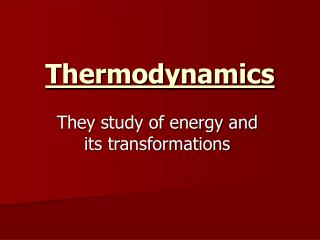Download PresentationThermodynamics

# Thermodynamics - PowerPoint PPT PresentationDownload Presentation## Thermodynamics

- - - - - - - - - - - - - - - - - - - - - - - - - - - E N D - - - - - - - - - - - - - - - - - - - - - - - - - - -
##### Presentation Transcript

1. Thermodynamics They study of energy and its transformations

2. Energy • Defined as the ability to do work or produce heat • 2 types of energy • Kinetic Energy – energy of motion (thermal energy or heat) • Potential Energy – energy of position (example – chemical bond energies) • Units – Joules = kg m2/sec2

3. First Law of Thermodynamics • – Energy is always conserved. Energy cannot be created or destroyed, it can only be converted into matter or another form of energy. E = mc2 • We must keep track of the energy exchanged in a process. So we divide the universe into two parts.

4. 2 Parts of the Universe Are . . . • The SYSTEM - part of the universe under study (generally a chemical process) • The SURROUNDINGS – the rest of the universe (like the container where a chemical reaction is taking place. • In chemistry we always focus on the SYSTEM!! • In a closed system - energy can be exchanged with the surroundings but not matter. • In an open system – energy and matter can be exchanged with the surroundings.

5. Internal Energy • Internal energy is the sum of the kinetic and potential energy of a system. • INTERNAL ENERGY = KE + PE

6. Internal Energy • We can only measure a change in energy (E) • E = Efinal – Einitial • It is a STATE FUNCTION – it only depends on current conditions. It does not matter how it got there.

7. Heat and Work • There are two ways for a system to exchange energy with its surroundings • Heat (q) = the amount of energy transferred between two objects • Work (w) = Energy that is a force acting over a distance w = F x d

8. Heat and Work • Heat and work are not state functions and are therefore dependent on the pathway. • The sum of heat and work = the change in Internal energy of a system E = q + w

9. Sign Conventions for work and heat • all thermodynamic variables include a number and a sign. • Heat (q) = (+) then heat isabsorbed by the system (surroundings cool down • Heat (q ) = (-) then heat is released from the system (and heats up the surroundings) • Work (w) = (+) then work is done on the system by the surroundings • Work (w) = (-) then work is done by the system on the surroundings

10. Change in Energy • E = (+) then the system gains energy • E = (-) then the system loses energy • Endothermic= energy is absorbed by the system by the surroundings as heat • Exothermic = energy is releasedby the system to the surroundings as heat.

11. Potential energy of product bonds are less than Reactants. • Products have stronger or more stable bonds • Potential Energy of the Products are higher than reactants. • Reactants have stronger more stable bonds.

12. Pressure Volume Work For chemical processes – work is done by a gas (through expansion) or work is done on a gas (by compression.) • Using P = force/area and w= force x d • you can derive work = PV • (P = external pressure) • For an expanding gas w = - PV • (work is being done on the surroundings)

13. Enthalpy • Enthalpy (H) is the amount of heat flow under conditions when only Pressure volume work is done. • PV work is only done under conditions of constant pressure with a volume expansion (+V) or volume compression (-V) H = E + PV

14. H = E + PV • (we also know E = qp + w) • Since E = qp - PV • (qp = heat at constant pressure) • H = qp + PV - PV so . . . . . • H = qp • Which means we can measure enthalpy by measuring the heat flow (or change in temperature) at constant pressure!

15. Heat at Constant Volumevs. Heat at Constant Pressure • Constant Volume • Bomb Calorimeter qv = ∆E Volume must change for work to be done, so no work term! • Constant Pressure • Coffee Cup Calorimeter – volume of a gas can change. qp = ∆H

16. Example -Hydrogen and oxygen gas are placed together in a container with a moveable piston, and the gases are ignited. As water is being produced, 1350 Joules of heat are lost to the surroundings. The gases expand, and the piston is moved, producing 350 Joules of work. What is the change in internal energy in this system? • Heat = q = -1350 J • Negative because the heat is lost to the surroundings – • Exothermic Reaction! • Work = w = -350 J • Negative because the work is done by the system • The piston, part of the system, is expanding and working against the surrounding pressure!

17. ∆E = q + w • = (-1350J) + (-350J) • = -1700 J of energy transferred to the surroundings. • Exothermic Process! Gas expands to keep constant pressure! H2 O2